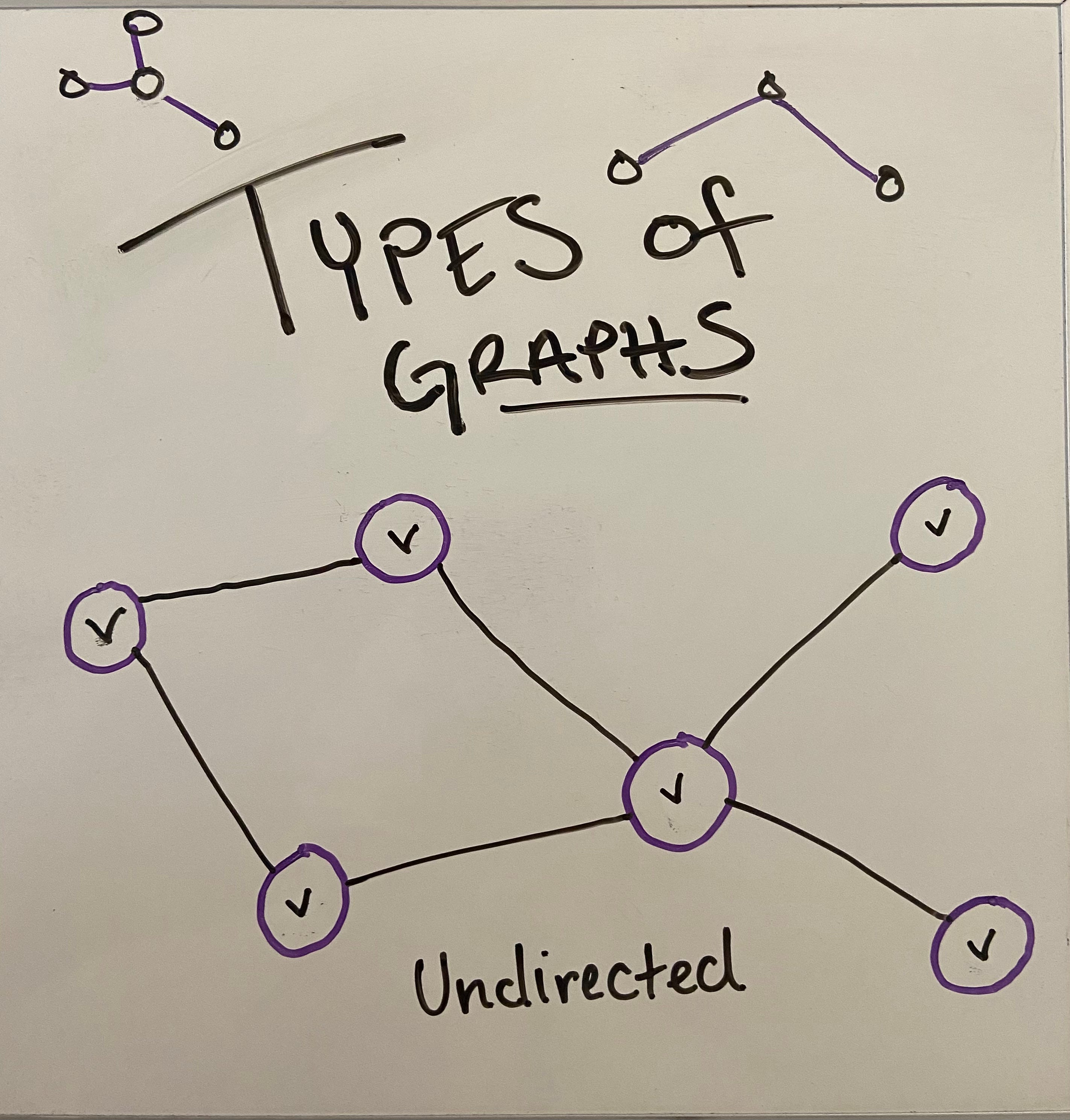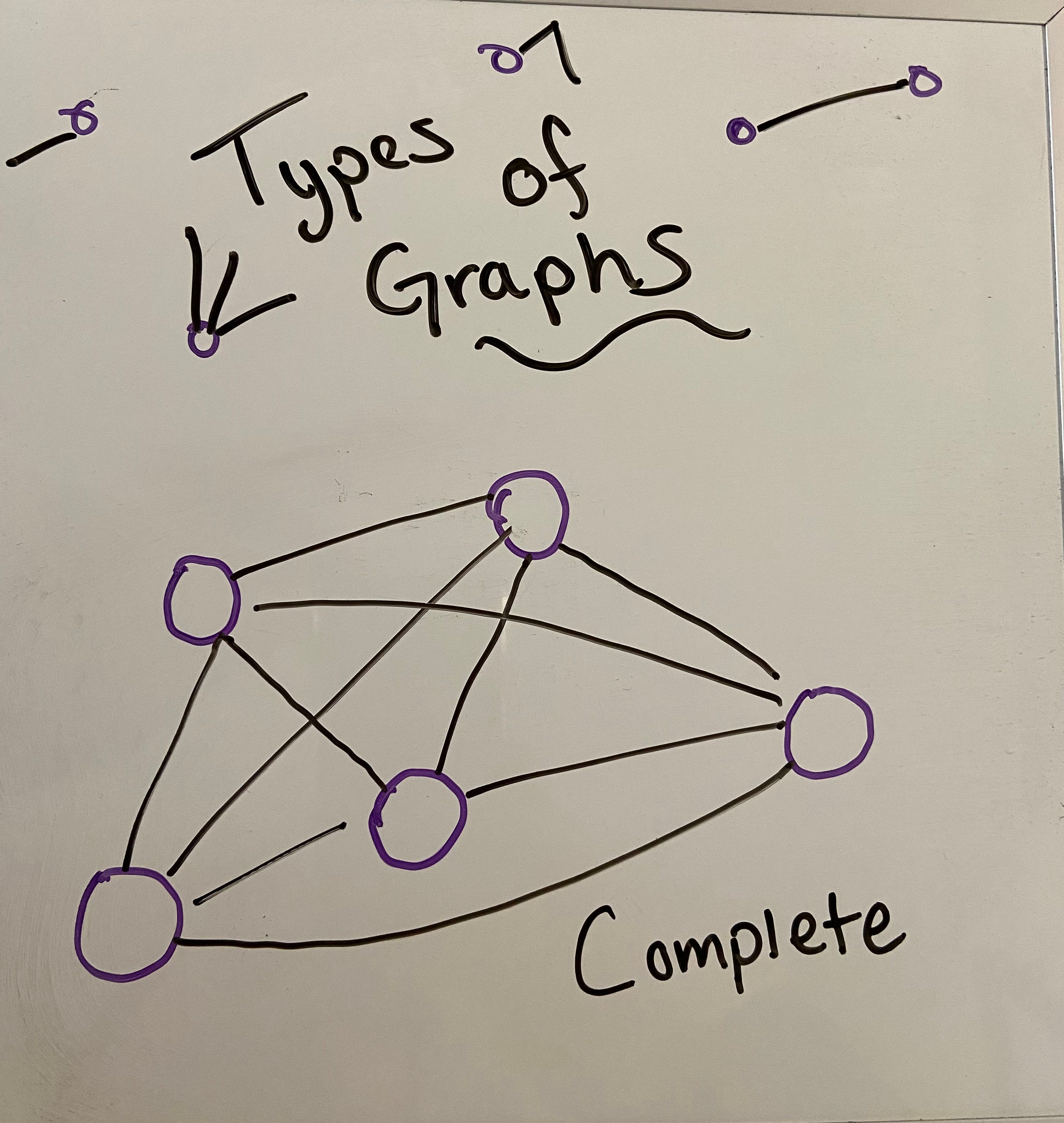# The Starting Line: Graphs in JavaScript

The Starting Line is a series in production by Roman Turner. Articles of helpful hints and technical tips that can jumpstart your journey into development. Written by a bootcamper, for bootcampers.

This is our first installment of a non-linear data structure. Much like how a Linked-List is a base structure that you give special methods and it evolves into a queue, or a stack, the Graph is a base structure that can be expounded upon.

So there is no confusion, for students in the United States, a Graph is often portrayed as a chart or a grid which is misleading in the proper terms of a Graph data structure. A graph as a data structure is depicted as a mess of nodes and connections.Photo by Clint Adair on Unsplash

# Grokking the Graph

There is a lot of content to talk about with Graphs, so I am going to break this up into a couple blog posts that will dive further into specific implementation and algorithmic examples for each. This post is on the big picture, an overview of the Graph.

## What is a Graph

“Graph data structure consists of a finite and possibly mutable set of vertices or nodes or points together with a set of ordered pairs of these vertices for an undirected graph or a set of ordered pairs for a directed graph.” — Wikipedia

To simplify the definition, a graph is just a set of nodes and their connections. Now, you might say, “a linked list fits that definition, it is a set of nodes that are connected.” That is true, but with a linked list, it has to be a linear-data structure. A graph on the other hand treats a node with indifference. There is no ‘entry’ point, there are just nodes and what links them together.
This puts it very simply, but until we start defining types of graphs we really just have a blob of nodes and pointers, imagine the nested holiday lights that live in a box in your parent’s attic.

## Graph Terminology

Let us start with using proper terminology when referencing a graph. All we know at this moment is that we have nodes and connections.

In a graph:

The node is referred to as a Vertex, many vertex are referred as Vertices.

The connections (link, pointer)between nodes is referred to as an Edge.

We are going to hold off on more terms, as there are many more but they can convolute us before we jump into what a graph is at its core.

# Graphs Represented in JavaScript

There are two common ways that a graph is represented in JavaScript; an Adjacency List, and an Adjacency Matrix.

## Adjacency Matrix

An adjacency matrix, which is a square matrix used to represent the edges of a graph.

“A square matrix is a two-dimensional array, an array which contains arrays all of equal size to itself. “ — Regina Furness

If you’d like to know more about implementing a weighted graph with an adjacency matrix checkout this blog:

A representation of a square matrix in JavaScript:

The matrix lists all nodes horizontally and vertically. With an adjacency matrix you can have a sparse graph or a dense graph depending on how many connections are made. You can also have a complete graph if all possible connections are made.

We are not going to dive in too deep into the adjacency matrix in this post but I will attach some references at the bottom of the blog for the curious.

## Adjacency List

The most common way to represent a graph, the adjacency list stores each vertex as a key in an Object that contains an array or linked-list of its edges. This is represented in JavaScript like this:

After looking at these representations doesn’t it help peel back the layers of confusing terms. They are simply keys and values of a primitive object that hold correlations that we can use to manipulate data with.

## More Terms

A Degree is the number of edges connected to a vertex (remember, because these are not linear structures they can have many edges).

Degree of a vertex: The total number of edges connected to a vertex. There are two types of degrees:

• In-Degree: The total number connected to a vertex.
• Out-Degree: The total of outgoing edges connected to a vertex.

Adjacency: Two vertices are said to be adjacent if there is an edge connecting them directly.

Parallel Edges: Two undirected edges are parallel​ if they have the same end vertices. Two directed edges are parallel if they have the same origin and destination.

Self Loop: This occurs when an edge starts and ends on the same vertex.

Isolated vertex: A vertex with zero degree.

Review these terms as you need them, but memorizing them at the moment isn’t needed to grok the graph.

# Types of Graphs

Here is where we will have many many different types of graphs and with that, many different use cases, morphing and verbiage.

## Graph Direction

The two typical suspects are undirected graphs , and directed graphs.Directed or Undirected Graphs

If the edges are multi or bi-directional, then we have an undirected graph.
If the edges have a direction, then we have a directed graph(di-graph). Much like a street you drive down, it could be a one-way (directed) or traffic flows both ways (undirected).

## Types of Graph

You will find that not all of the nodes will be connected. There are special names for each type of graph based on the ‘connectedness’ (made that up just now) of the graph.Connected/Disconnected/Complete Graphs

Starting with all vertex that share at least one edge with another vertex is a Connected Graph (I admit while writing this section I called each vertex a node, and had to go back and change it).

When you have a graph where you have an isolated vertex, you have a Disconnected Graph.

And the messy but Complete Graph where every vertex shares an edge with every other vertex. You can compute this easily though where a complete graph each node should have the amount of vertex-1 edges.

Now, edges can also hold a value. When the edge holds a value it is referred to as its weight. When a graph has weighted edges, it is referred to as a Weighted Graph.

# Whew, you made it.

Hopefully this sparked some understanding, and made some gears click for you with the Graph data structure. This is a part of the The Starting Line: Data Structure Skim a weekly article series where we will explore the wonderful things that hold wonderful things and resources on how to work with them.

In my next Starting Line post I will go through a graph implementation with an Adjacency List and some common use cases with an algorithm example from Leetcode. First we understand the what, then the why and how ☺️

Additional Resources:

Implementation of Graphs

Intro to Graphs

Common Graph Algorithms

Seattle Software Engineer. A collector of new skills, with a fondness for all things sushi.

## More from Roman Turner

Seattle Software Engineer. A collector of new skills, with a fondness for all things sushi.